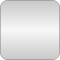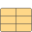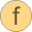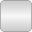# OneNonLinearEquation

Determine solution of a non-linear algebraic equation in one unknown without derivatives in a reliable and efficient way# Package Contentsf_nonlinear_Data Data specific for function f_nonlinearf_nonlinear Nonlinear algebraic equation in one unknown: y = f_nonlinear(x,p,X)solve Solve f_nonlinear(x_zero)=y_zero; f_nonlinear(x_min) - y_zero and f_nonlinear(x_max)-y_zero must have different sign

# Information

This information is part of the Modelica Standard Library maintained by the Modelica Association.

This function should currently only be used in Modelica.Media, since it might be replaced in the future by another strategy, where the tool is responsible for the solution of the non-linear equation.

This library determines the solution of one non-linear algebraic equation "y=f(x)" in one unknown "x" in a reliable way. As input, the desired value y of the non-linear function has to be given, as well as an interval x_min, x_max that contains the solution, i.e., "f(x_min) - y" and "f(x_max) - y" must have a different sign. If possible, a smaller interval is computed by inverse quadratic interpolation (interpolating with a quadratic polynomial through the last 3 points and computing the zero). If this fails, bisection is used, which always reduces the interval by a factor of 2. The inverse quadratic interpolation method has superlinear convergence. This is roughly the same convergence rate as a globally convergent Newton method, but without the need to compute derivatives of the non-linear function. The solver function is a direct mapping of the Algol 60 procedure "zero" to Modelica, from:

Brent R.P.:
Algorithms for Minimization without derivatives. Prentice Hall, 1973, pp. 58-59.

Due to current limitations of the Modelica language (not possible to pass a function reference to a function), the construction to use this solver on a user-defined function is a bit complicated (this method is from Hans Olsson, Dassault Systèmes AB). A user has to provide a package in the following way:

```  package MyNonLinearSolver
extends OneNonLinearEquation;

redeclare record extends Data
// Define data to be passed to user function
...
end Data;

redeclare function extends f_nonlinear
algorithm
// Compute the non-linear equation: y = f(x, Data)
end f_nonlinear;

// Dummy definition that has to be present for current Dymola
redeclare function extends solve
end solve;
end MyNonLinearSolver;

x_zero = MyNonLinearSolver.solve(y_zero, x_min, x_max, data=data);
```

# Extended by (1)Inverse_sine_definition Modelica.Media.Examples.SolveOneNonlinearEquation.Inverse_sine Define sine as non-linear equation to be solved